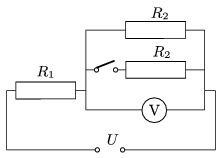Mathematical and Physical Journal
for High Schools
Issued by the MATFUND Foundation
 Already signed up? New to KöMaL?

#Problem P. 4505. (January 2013)

P. 4505. The values the resistance of the resistors connected to the voltage supply of U=120 V, as shown in the figure are unknown. When the switch is closed the reading on the voltmeter changes by 16 V, and the power dissipated by the resistor R1 changes by 51.2 W.a) Using the condition given to the change in the voltage determine the ratio of the resistances: R1/R2.

b) What are the values of the resistance of the resistors R1 and R2?

(4 pont)

Deadline expired on February 11, 2013.

Sorry, the solution is available only in Hungarian. Google translation

Megoldásvázlat. $\displaystyle a)$ $\displaystyle R_1/R_2=2$ vagy 1/4.

$\displaystyle b)$ $\displaystyle R_1=55~\Omega$ és $\displaystyle R_2=27{,}5~\Omega$ vagy $\displaystyle R_1=20~\Omega$ és $\displaystyle R_2=80~\Omega$.

### Statistics:

 80 students sent a solution. 4 points: 54 students. 3 points: 17 students. 2 points: 6 students. 1 point: 3 students.

Problems in Physics of KöMaL, January 2013